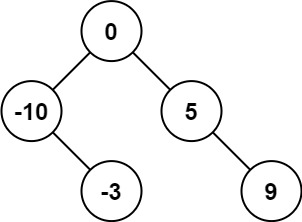# Convert Sorted Array to Binary Search Tree LeetCode Programming Solutions | LeetCode Problem Solutions in C++, Java, & Python [💯Correct]

LeetCode Problem | LeetCode Problems For Beginners | LeetCode Problems & Solutions | Improve Problem Solving Skills | LeetCode Problems Java | LeetCode Solutions in C++

Hello Programmers/Coders, Today we are going to share solutions to the Programming problems of LeetCode Solutions in C++, Java, & Python. At Each Problem with Successful submission with all Test Cases Passed, you will get a score or marks and LeetCode Coins. And after solving maximum problems, you will be getting stars. This will highlight your profile to the recruiters.

In this post, you will find the solution for the Convert Sorted Array to Binary Search Treein C++, Java & Python-LeetCode problem. W e are providing the correct and tested solutions to coding problems present on LeetCode. If you are not able to solve any problem, then you can take help from our Blog/website.

`Use “Ctrl+F” To Find Any Questions Answer. & For Mobile User, You Just Need To Click On Three dots In Your Browser & You Will Get A “Find” Option There. Use These Option to Get Any Random Questions Answer.`

LeetCode is one of the most well-known online judge platforms to help you enhance your skills, expand your knowledge and prepare for technical interviews.

LeetCode is for software engineers who are looking to practice technical questions and advance their skills. Mastering the questions in each level on LeetCode is a good way to prepare for technical interviews and keep your skills sharp. They also have a repository of solutions with the reasoning behind each step.

LeetCode has over 1,900 questions for you to practice, covering many different programming concepts. Every coding problem has a classification of either EasyMedium, or Hard.

LeetCode problems focus on algorithms and data structures. Here is some topic you can find problems on LeetCode:

• Mathematics/Basic Logical Based Questions
• Arrays
• Strings
• Hash Table
• Dynamic Programming
• Stack & Queue
• Trees & Graphs
• Greedy Algorithms
• Depth-First Search
• Sorting & Searching
• BST (Binary Search Tree)
• Database
• Recursion, etc.

Leetcode has a huge number of test cases and questions from interviews too like Google, Amazon, Microsoft, Facebook, Adobe, Oracle, Linkedin, Goldman Sachs, etc. LeetCode helps you in getting a job in Top MNCs. To crack FAANG Companies, LeetCode problems can help you in building your logic.

Link for the ProblemConvert Sorted Array to Binary Search Tree– LeetCode Problem

`Convert Sorted Array to Binary Search Tree– LeetCode Problem`

### Problem:

Given an integer array `nums` where the elements are sorted in ascending order, convert it to a height-balanced binary search tree.

height-balanced binary tree is a binary tree in which the depth of the two subtrees of every node never differs by more than one.

Example 1:

```Input: nums = [-10,-3,0,5,9]
Output: [0,-3,9,-10,null,5]
Explanation: [0,-10,5,null,-3,null,9] is also accepted:```

Example 2:

```Input: nums = [1,3]
Output: [3,1]
Explanation: [1,3] and [3,1] are both a height-balanced BSTs.
```

Constraints:

• `1 <= nums.length <= 104`
• `-104 <= nums[i] <= 104`
• `nums` is sorted in a strictly increasing order.
`Convert Sorted Array to Binary Search Tree– LeetCode Solutions`
`Convert Sorted Array to Binary Search Tree Solution in C++:`
```class Solution {
public:
TreeNode* sortedArrayToBST(vector<int>& nums) {
return helper(nums, 0, nums.size() - 1);
}

private:
TreeNode* helper(vector<int>& nums, int l, int r) {
if (l > r)
return nullptr;

const int m = l + (r - l) / 2;
TreeNode* root = new TreeNode(nums[m]);
root->left = helper(nums, l, m - 1);
root->right = helper(nums, m + 1, r);

return root;
}
};```
`Convert Sorted Array to Binary Search Tree Solution in Java:`
```class Solution {
public TreeNode sortedArrayToBST(int[] nums) {
return helper(nums, 0, nums.length - 1);
}

private TreeNode helper(int nums[], int l, int r) {
if (l > r)
return null;

final int m = l + (r - l) / 2;
TreeNode root = new TreeNode(nums[m]);
root.left = helper(nums, l, m - 1);
root.right = helper(nums, m + 1, r);

return root;
}
}```
`Convert Sorted Array to Binary Search Tree Solution in Python:`
```class Solution:
def sortedArrayToBST(self, nums: List[int]) -> TreeNode:
def helper(l: int, r: int) -> TreeNode:
if l > r:
return None

m = (l + r) // 2
root = TreeNode(nums[m])
root.left = helper(l, m - 1)
root.right = helper(m + 1, r)

return root

return helper(0, len(nums) - 1)```